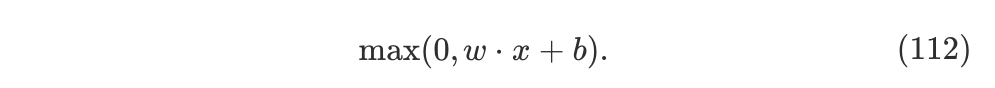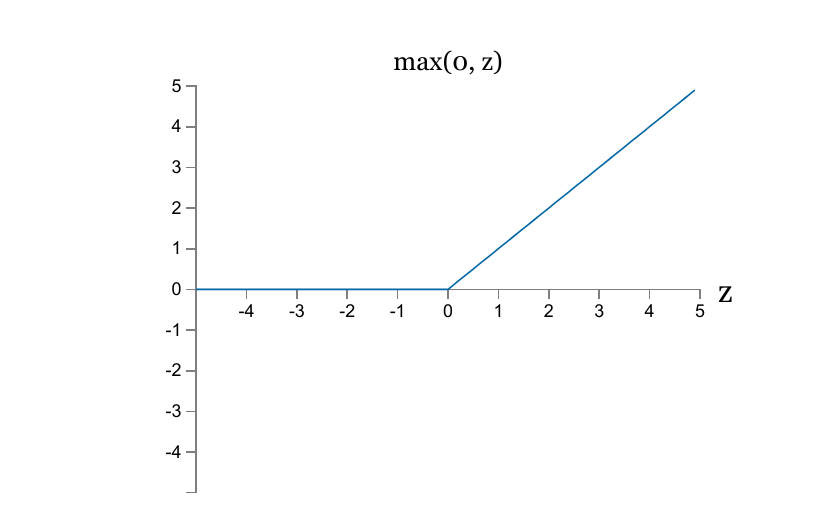ch-3 Improving the way neural networks learn

The techniques we’ll develop in this chapter include

• a better choice of cost function, the cross-entropy cost function;
• four so-called “regularization” methods ( L1 and L2 regularization, dropout, and artificial expansion of the training data ), which make our networks better at generalizing beyond the training data;
• a better method for initializing the weights in the network;
• a set of heuristics to help choose good hyper-parameters ;

The cross-entropy cost function

Problem of quadratic function

Artificial neuron has a lot of difficulty learning when it’s badly wrong

w = b = 2.0, η = 0.15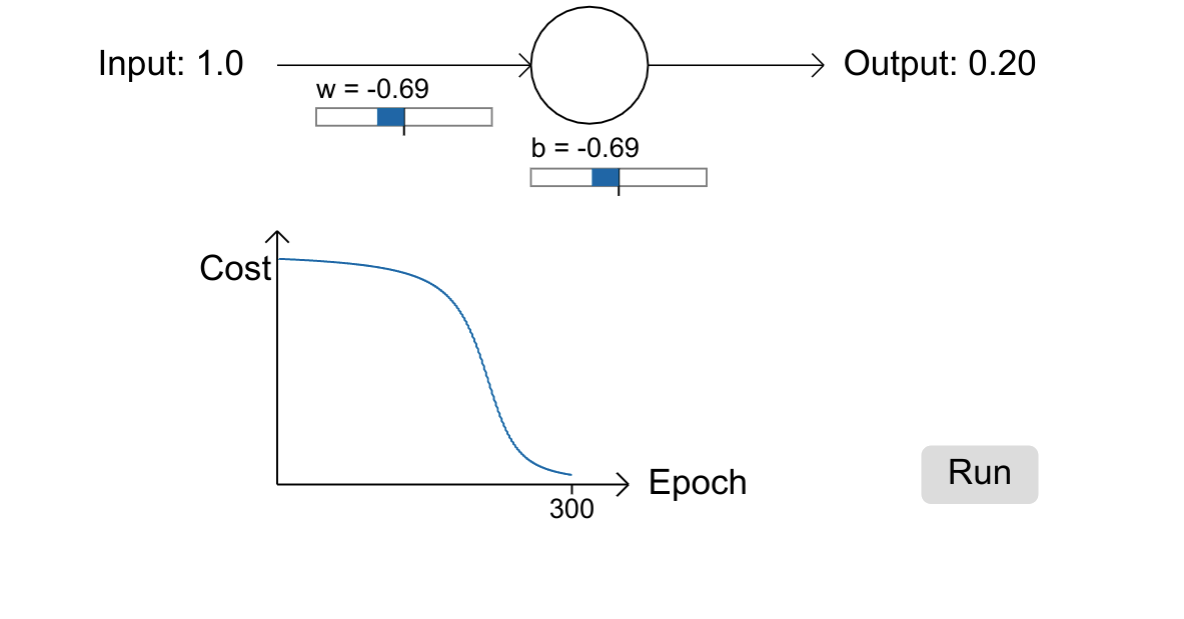Quadratic cost function $C = \frac { (y-a) ^ 2 }{ 2 }$• when the neuron’s output is close to 1 ( might be far away from the desired output )
• the curve gets very flat, so $σ′(z)$ gets very small.
• so $∂C/∂w$ and $∂C/∂b$ get very small.

Introducing cross-entropywhere $a = σ(z)$ and $z= \Sigma_j w_jx_j + b$

1. It’s a cost function :
• non-negative
• close to zero when a close to y
1. avoids the problem of learning slowing down
• rates not controlled by $σ’(z)$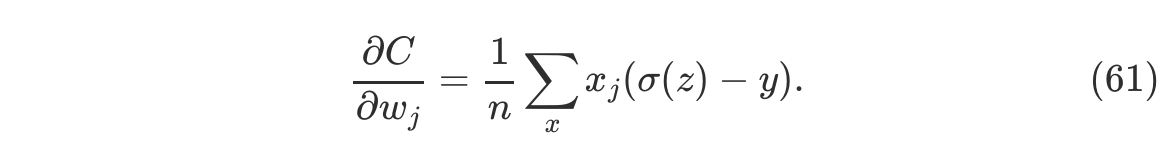Using the quadratic cost when we have linear neurons in the output layer

• all the neurons in the final layer are linear neurons
• outputs are simply $a_j^L = y_j^L$, sigmoid not applied
• quadratic cost will not slowdown learning

Softmax

• define a new type of output layer
• apply the so-called softmax function to zoutput is a set of positive numbers which sum up to 1, can be thought of as a probability distribution

The learning slowdown problem

log-likelihood cost functionprobability of $a_y^L$ close to 1, cost close to 0

$δ_j ^L = \partial C / \partial z_j^L$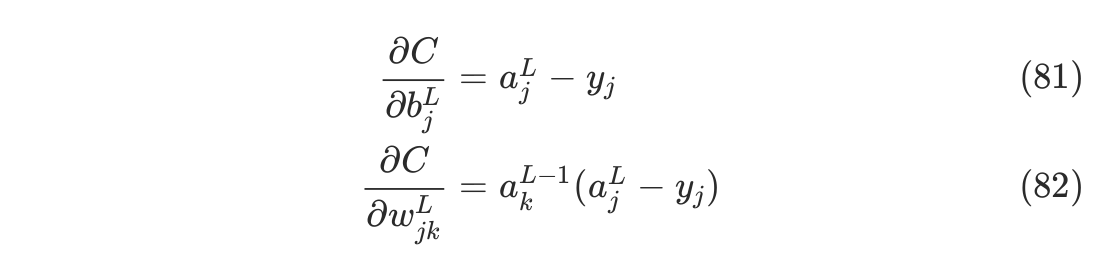Overfitting

cost on the training data continue decrease while

classification accuracy on the test data stops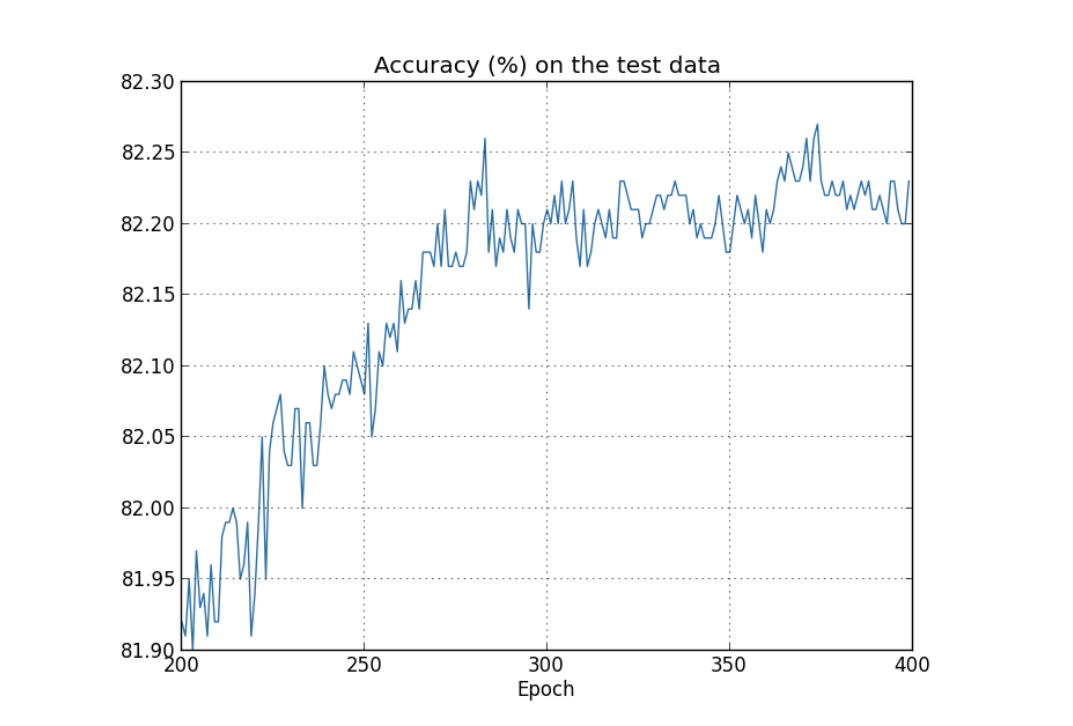cost on the test data starts increase while

classification accuracy on training data rises up to 100%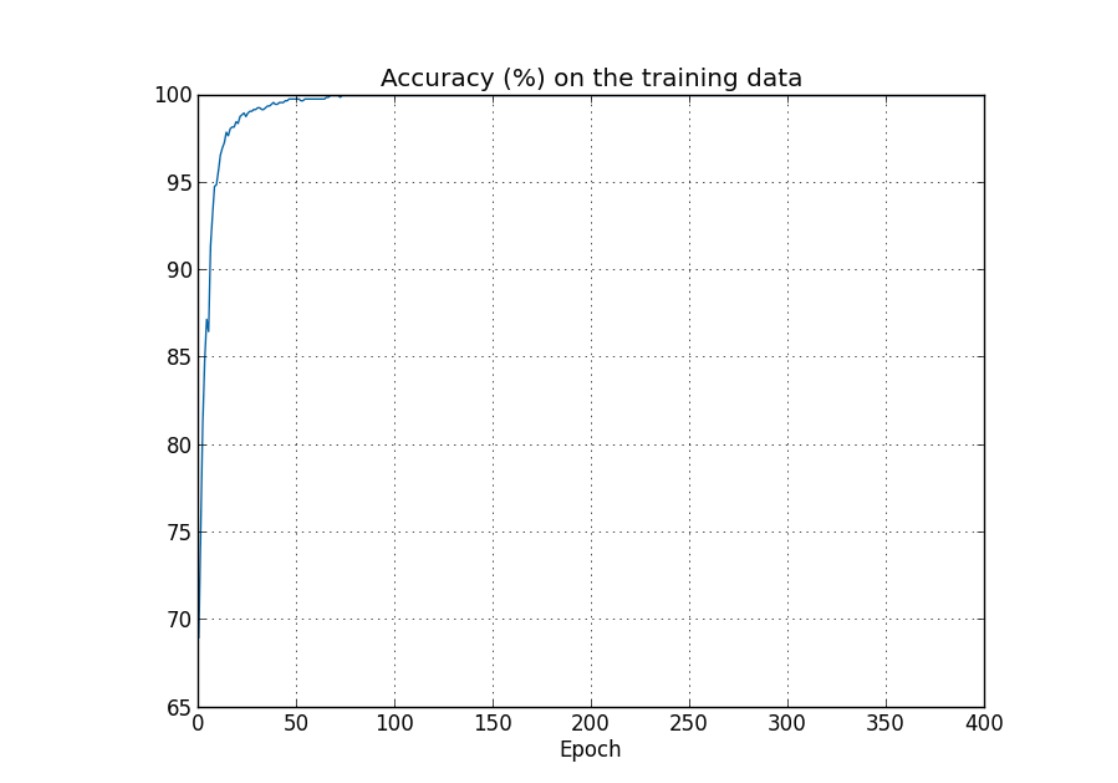It’s almost as though our network is merely memorizing the training set, without understanding digits well enough to generalize to the test set.

Detect overfitting

• keeping track of accuracy on the test data as our network trains.
• using validation data: we may find hyper-parameters which fit particular peculiarities of the test_data if using test_data for detection.
• early stopping: stop training when accuracy no longer improving
• hold out method: validation_data is kept apart or “held out” from the training_data

Avoid overfitting

• one of the best ways: increase the size of the training data
• regularization

Regularization

L2 regularization ( weight decay )

add an extra term on cost function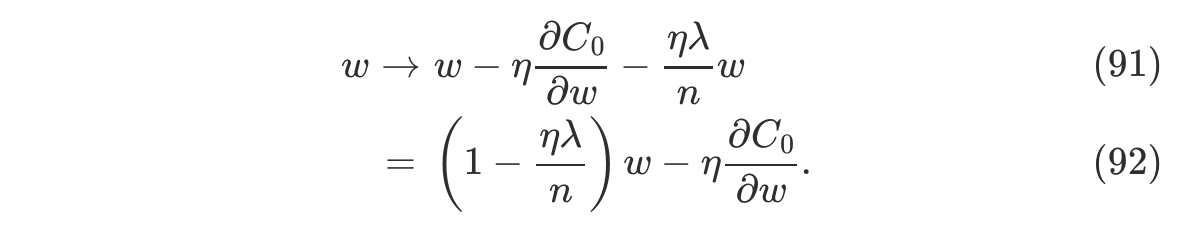• Intuitively, the effect of regularization is to make it so the network prefers to learn small weights
• λ : compromising between finding small weights and minimizing the original cost function
• $-\frac{ηλ}{n}*w$ weights shrink by an amount proportional to w

Why does regularization help reduce overfitting?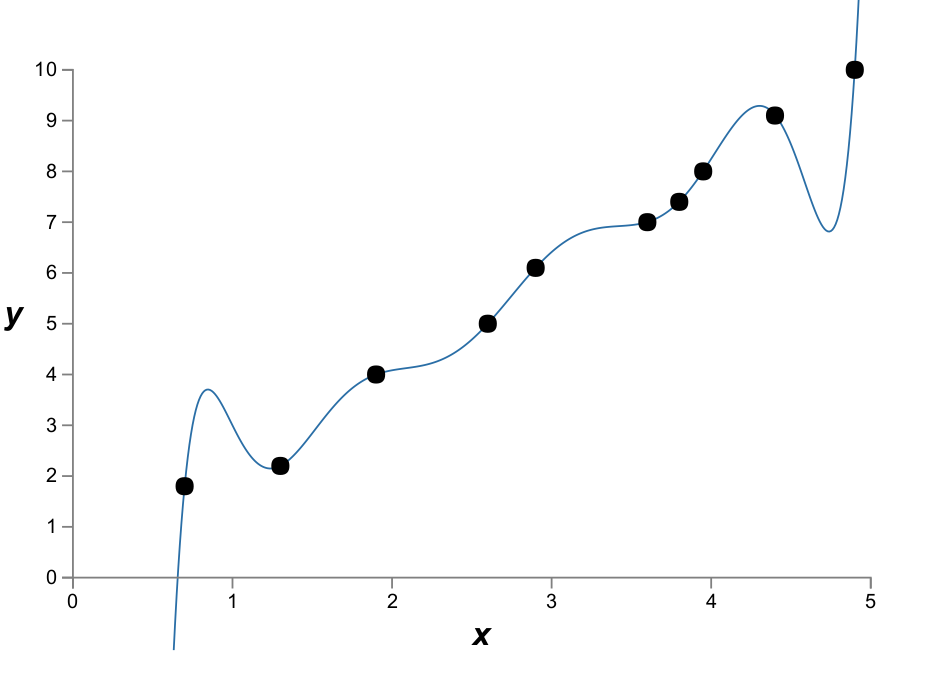the 9th order model is really just learning the effects of local noise

• The smallness of the weights means that the network won’t change too much if we change a few random inputs here and there
• having a large bias doesn’t make a neuron sensitive to its inputs in the same way as having large weights

We don’t have an entirely satisfactory systematic understanding of what’s going on, merely incomplete heuristics and rules of thumb.

L1 regularization• $-\frac{ηλ}{n} * sgn(w)$weights shrink by a constant amount toward 0
• when $|w|$ is large, shrink less; when $|w|$ is small, shrink more

Dropout

modify the network itself rather than modifying the cost function

• start by randomly deleting half the hidden neurons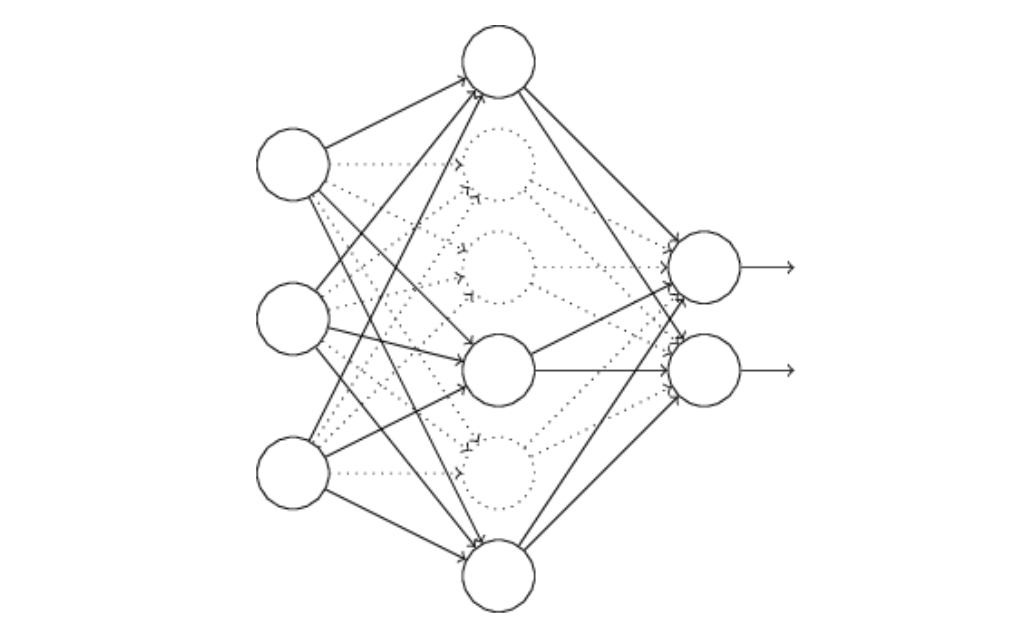• forward-propagate the input and backpropagate the result, update network
• choosing a new random subset, repeat;
• Finally, run the full network by halving all weights.

Heuristic

• Averaging the effects of a large number of different networks
• a neuron cannot rely on particular other neurons, robust to losing any individual connection

Artificially expanding the training data

The general principle is to expand the training data by applying operations that reflect real-world variation.

An aside on big data and what it means to compare classification accuracies

more training data can sometimes compensate for differences in the machine learning algorithm used

Weight initialization

Up to now

• choose w & b using independent Gaussian random variables
• mean 0 and standard deviation 1
• while z sum up over a total of 501 normalized Gaussian random variables
• z will have a very broad Gaussian distribution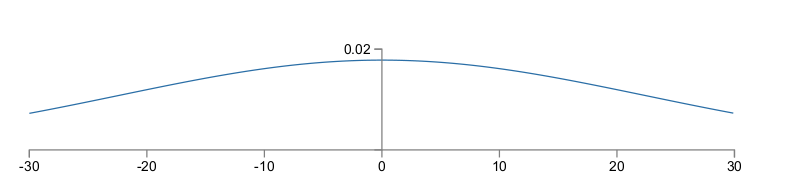An easy way

• initialize more sharply peak
• mean 0 and standard deviation $1 / \sqrt {n_{in}}$
• seems only speeds up learning, doesn’t change the final performance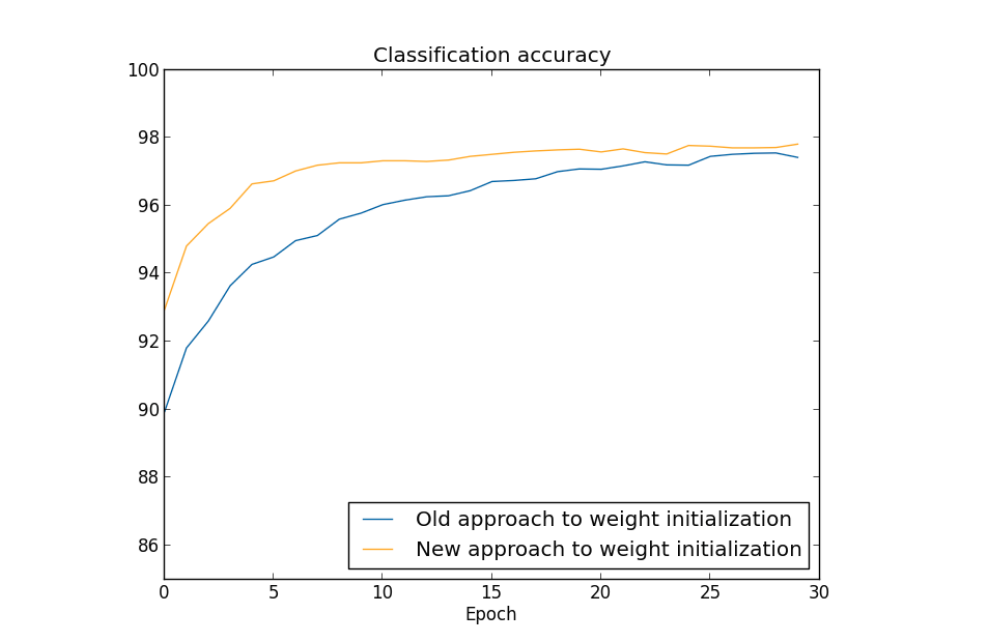Connection with Regularization

• L2 regularization sometimes automatically gives us something similar to the new approach to weight initialization

How to choose a neural network’s hyper-parameters?

Problem when choose η=10.0 and λ=1000.0

• classification accuracies are no better than chance

• examine network to achieve results better than chance

Speed up experimentation

• stripping network down to the simplest
• increasing the frequency of monitoring

When having a signal

• gradually decrease the frequency of monitoring
• experiment with a more complex architecture, adjust η and λ again

Learning Rate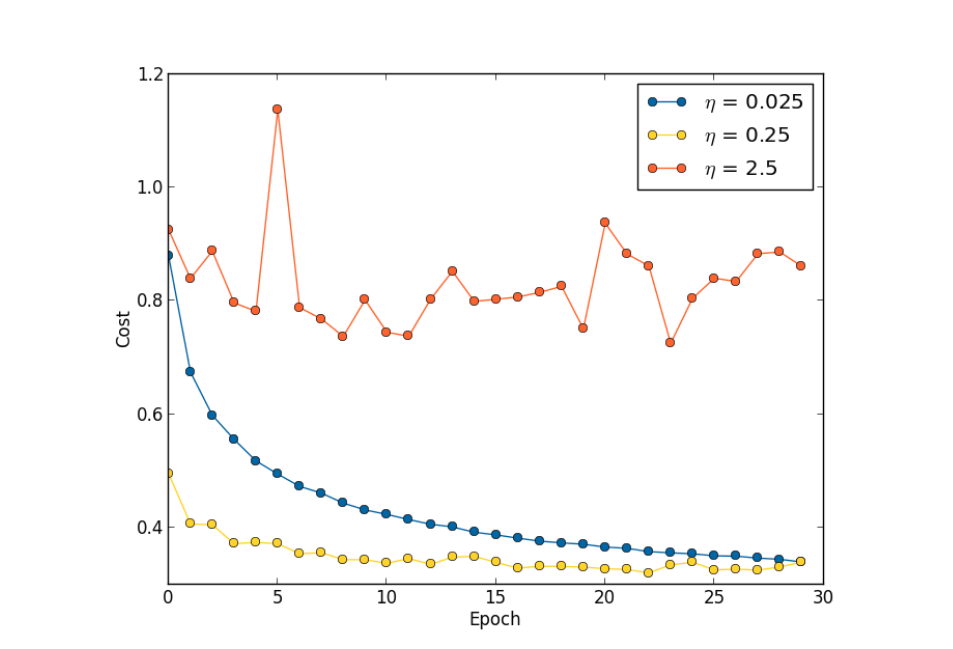• First estimate the order of threshold for η so that training cost won’t oscillating or increasing
• control the step size in gradient descent, no need to monitor by accuracy

Number of training Epochs

Early stopping

• terminate when classification on validation data stops improving

Learning rate schedule

• μ=0 there’s a lot of friction, the velocity can’t build upuse a large learning rate when weights are badly wrong
• later reduce as we make more fine-tuned adjustments

The regularization parameter, λ

• starting initially λ=0.0 to determine η
• increase or decrease by factors of 10, get a fine-tune
• return and re-optimize η again

Mini-batch size

• it’s possible to use matrix techniques to compute the gradient update for all examples in a mini-batch simultaneously
• using the larger mini-batch would speed things up

Other techniques

####Variations on stochastic gradient descent

Hessian technique

incorporates not just the gradient, but also information about how the gradient is changing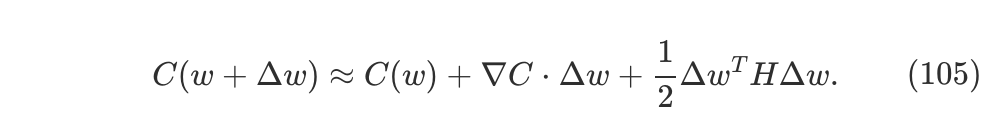$H$ is a matrix known as the Hessian matrix, whose $jkth$ entry is $∂^2C/∂w_j∂w_k$• the sheer size of the Hessian matrix make it difficult to compute

introduces a notion of “velocity” and “friction”

• replace the gradient descent update rule $w→w′=w−η∇C$ by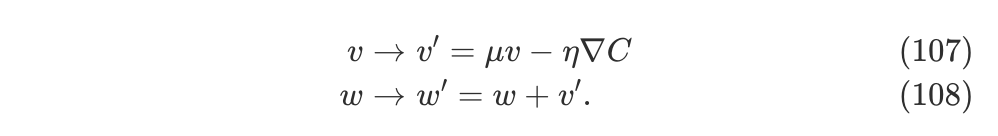• the “force” ∇C is now modifying v, and the velocity is controlling the rate of change of w.
• 1−μ as the amount of friction in the system.
• μ=1, there is no friction; μ=0 there’s a lot of friction, the velocity can’t build up;

Other models of artificial neuron

tanh neuron

• ranges from -1 to 1, not 0 to 1
• allows both positive and negative activations
• the activations in hidden layers would be equally balanced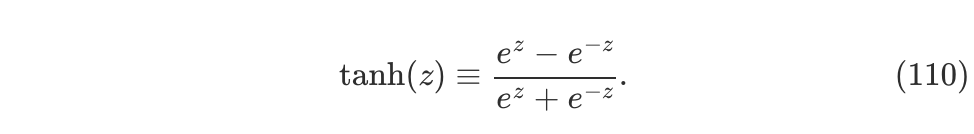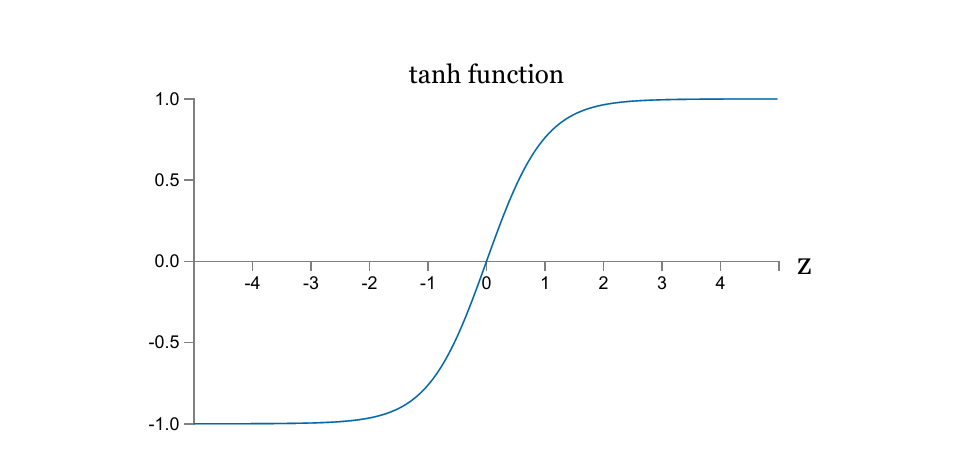Rectified linear unit

• never cause saturate, no corresponding learning slowdown